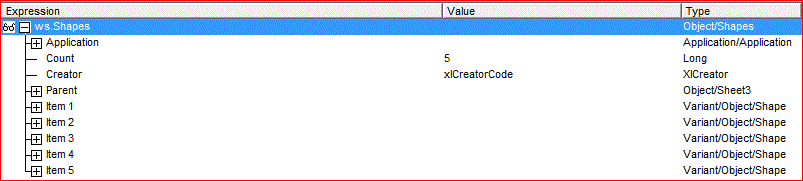## Situation

Allen's most recent email had a user question regarding how to change the text in a textbox of a chart.

"Easy cheesy, I thought, "I've already written this up!"  (See Changing the value in a worksheet text box, Parts 1, 2, and 3).

Well, smarty-pants, there's always something new to learn, so I read the question more carefully.  I started wondering if there was such a thing as a "textbox of a chart", meaning a textbox that has been added to a chart.  After all, the workbook I was working with up to this time was developed with pre-Excel 2007 software.

## Discovery

Sure enough, with a little experimentation I found that a textbox could be linked to a chart.

### Experiment Set-up

My work doesn't call for many charts, so I created a simple table with first of the month for a year in column A and random numbers between 1 and 50 in column B.  Because this person mentioned he had multiple charts on the page, I added two charts, one a bar chart and the other a line chart.  He also mentioned each chart had two Text Boxes.Figure 1 - Worksheet with Charts and Text Boxes

### Examination

As my usual method for understanding the object model, I ran a small sub that simply assigned the worksheet, then examined the breakdown, focusing on the Shapes, where I knew the Charts could be found.

I expected to find either three shapes (the two Charts and the orange text box) or seven,Figure 2 - Shapes with the Worksheet as the Parent

Instead, as shown above, there were five.  That is because, driving down, I found there are 2 Shapes within Item2, which is "Chart 3".Figure 3 - Shapes with a Chart as the Parent

## Making the Discovery Useful

### A Priori Knowledge

Something to remember is that the information shown is not directly on the Shape object.
• Like a Chart object is a property of a Shape where the Type is msoChart, when Shape.Type = msoTextBox we must look into the DrawingObject property of the Shape contains the value shown in the Text Box.
• The  value shown is in DrawingObject.Caption.

### Refinement

Previously I noted how to assign a value in VBA.  Now there are two possibilities.
Worksheets("Sheet1").Shapes("Text Box 3").DrawingObject.Caption = <String>
Worksheets("Sheet1").Shapes("Chart 2").Chart.Shapes("Text Box 4").DrawingObject.Caption =  <String>

### Development Support Tool

A common exercise in how-to books is to roll through part of the object model to extract information that can then be used for further development and documenting the solution.  The following Sub, attached to a Worksheet called "TextBoxes" can be used to identify the names of all of the text boxes and their parents.
Sub InventoryChartsAndTextBoxes()
Const wsInvName As String = "TextBoxes"
Dim wsInv As Worksheet
Dim ws As Worksheet
Dim sh1 As Shape
Dim sh2 As Shape
Dim dro As Object
Dim RowNum As Double
Set wsInv = ThisWorkbook.Worksheets(wsInvName)
wsInv.UsedRange.Rows.Delete
wsInv.Cells(1, 1) = "Worksheet Name"
wsInv.Cells(1, 2) = "Lev 1 Shape Name"
wsInv.Cells(1, 3) = "Lev 1 Shape Type"
wsInv.Cells(1, 4) = "Lev 1 Caption"
wsInv.Cells(1, 5) = "Lev 2 Shape Name"
wsInv.Cells(1, 6) = "Lev 2 Shape Type"
wsInv.Cells(1, 7) = "Lev 2 Caption"
RowNum = 1
For Each ws In ThisWorkbook.Worksheets
For Each sh1 In ws.Shapes
Select Case sh1.Type
Case msoChart
RowNum = RowNum + 1
wsInv.Cells(RowNum, 1) = ws.Name
wsInv.Cells(RowNum, 2) = sh1.Name
wsInv.Cells(RowNum, 3) = "Chart" ' sh1.Type
For Each sh2 In sh1.Chart.Shapes
If sh2.Type = msoTextBox Then
RowNum = RowNum + 1
wsInv.Cells(RowNum, 1) = ws.Name
wsInv.Cells(RowNum, 2) = sh1.Name
wsInv.Cells(RowNum, 3) = "Chart"
wsInv.Cells(RowNum, 5) = sh2.Name
wsInv.Cells(RowNum, 6) = "Text Box"
wsInv.Cells(RowNum, 7) = sh2.DrawingObject.Caption
End If
Next sh2
Case msoTextBox
RowNum = RowNum + 1
wsInv.Cells(RowNum, 1) = ws.Name
wsInv.Cells(RowNum, 2) = sh1.Name
wsInv.Cells(RowNum, 3) = "Text Box"
wsInv.Cells(RowNum, 4) = sh1.DrawingObject.Caption
End Select
Next sh1
Next ws
End Sub